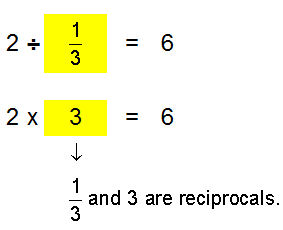# Multiply and Divide Fractions and Mixed Numbers

Students learn how to multiply fractions with and without cancelling, multiply mixed numbers, and the meaning of reciprocals. They also learn how to divide fractions, divide mixed numbers, and to solve real-world problems. There are 10 in-depth lessons in this unit, aligned to the Common Core.Lessons on Fractions Multiplying Fractions Multiplying Fractions by Cancelling Common Factors Multiplying Mixed Numbers Reciprocals Dividing Fractions Dividing Mixed Numbers Solving Word Problems Practice Exercises Challenge Exercises Solutions